| Technical Review: Aaditya Damle and ABCOM Team | Copy Editor: Anushka Devasthale | Last Updated: Aug 13, 2020 | Level: Beginner | Banner Image Source Internet |Image Source Internet

# Introduction

Logistic Regression is a statistical method of classification of objects. What does classification mean? Let me take a few examples to illustrate what we mean by classification. A doctor classifies the tumor as malignant or benign. A bank transaction may be fraudulent or genuine. For many years, humans have been performing such tasks - albeit they are error-prone. Can we train machines to do these tasks for us with better accuracy? One such example of a computer doing the classification is the email Client on your device that classifies every incoming mail as “spam” or “not spam.” It does it with relatively high accuracy. The statistical technique of logistic regression has been successfully applied in email clients. That is to say; we have trained our machine to solve a classification problem. Logistic Regression is just one part of machine learning used for solving this kind of binary classification problem. Several other machine learning techniques are already developed and are in practice for solving different types of issues.

In all the above examples, the prediction outcome has only two values - Yes or No. We call these as classes - to say, we say that our classifier classifies the objects in two classes. In technical terms, we say that the outcome or target variable is dichotomous. There are other classification problems in which the output may be classified into more than two classes For example, given a basket full of fruits, you are asked to separate different kinds of fruits. Now, the basket may contain Oranges, Apples, Mangoes, and so on. So, when you separate out the fruits, you separate them in more than two classes. This example is a multivariate classification problem.

In this tutorial, we will focus on solving binary classification problems using logistic regression techniques. I will take a specific case study and code for it to learn how to apply logistic regression practically. I assume your familiarity with Python and many of its libraries, such as Pandas, NumPy, and matplotlib. Next, I will describe the case study.

## Case study

Consider a bank that approaches you to develop a machine learning application that will help them identify potential clients who would open a Term Deposit (also called Fixed Deposit by some banks) with them. The bank regularly surveys through telephonic calls or web forms to collect information about potential clients. The survey is of a general kind and is conducted over a vast audience, out of which many may not be interested in dealing with this bank itself. Out of the rest, only a few may be interested in opening a Term Deposit. Others may be interested in other facilities offered by the bank. So, the survey is not necessarily conducted for identifying the customers opening TDs. Your task is to identify all those customers with a high probability of opening TD from the humongous survey data that the bank will share with you.

Fortunately, one such kind of data is publicly available for those aspiring to develop machine learning models. Some students prepared this data at UC Irvine with external funding. The database is available as a part of the UCI Machine Learning Repository and is widely used by students, educators, and researchers worldwide. The data can be downloaded from the UCI repository.

Let us now begin the application development:

# Setting up Project

## Installing Jupyter

We will be using Jupyter - one of the most widely used platforms for machine learning. If you do not have Jupyter installed on your machine, download it from the Jupyter site. Follow the instructions on their site to install the platform. As the website suggests, you may prefer to use Anaconda Distribution, which comes with Python and many commonly used Python packages for scientific computing and data science. This will alleviate the need to install these packages individually.

After the successful installation of Jupyter, start a new project. Your screen at this stage would look like the following ready to accept your code.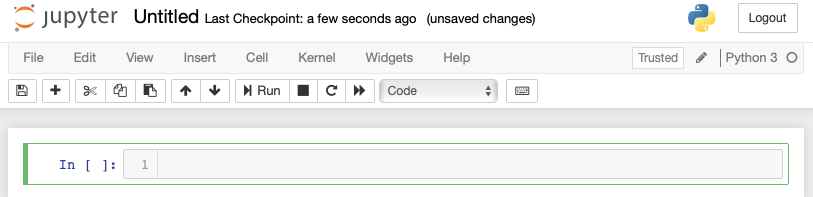Change the name of the project from Untitled1 to Logistic Regression by clicking on the title name and editing it.

First, we will be importing several Python packages that we will need in our code.

## Importing Python Packages

Type or cut-n-paste following code in the code editor:

``````#import statements
import pandas as pd
import numpy as np
import matplotlib.pyplot as plt

from sklearn import preprocessing
from sklearn.linear_model import LogisticRegression
from sklearn.model_selection import train_test_split
``````

Your Notebook should look like the following at this stage: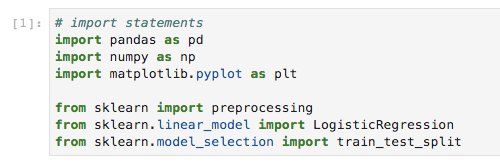Run the code by clicking on the Run button. If no errors are generated, you have successfully installed Jupyter and are ready for the rest of the development.

The first three import statements import pandas, numpy, and matplotlib.pyplot packages in our project. The next statements import the three specified modules from sklearn.

# Getting Data

If you have not already downloaded the UCI dataset mentioned earlier, download it from the UCI Machine Learning repository. Click on the Data Folder. You will see the following screen: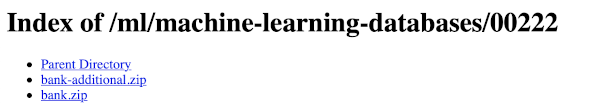Download the bank.zip file by clicking on the given link. The zip file contains the following files: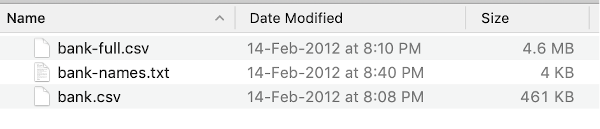We will use the bank-full.csv file for our model development. The bank-names.txt file contains the description of the database that you are going to need later. The bank.csv is smaller than bank-full.csv, which contains a larger dataset that you may use for more advanced developments.

Please copy the bank-full.csv file in your project folder and proceed with the next step of development.

To load the data from the CSV file that you copied just now, type the following statement and run it.

``````df = pd.read_csv('bank-full.csv', sep = “;”, header=0)
``````

You will be able to examine the loaded data by running the following code statement:

``````df.head()
``````

You will see the following output: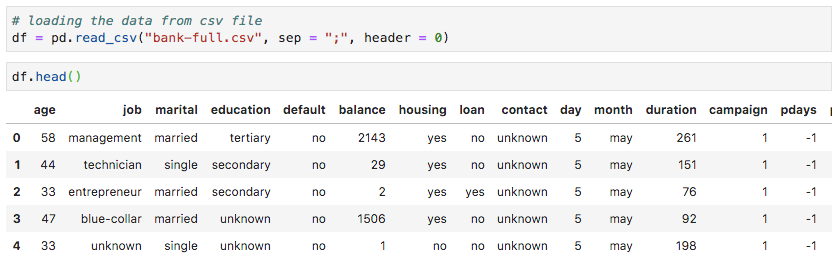It has printed the first five rows of the loaded data. There are 21 columns. Examine them. We will be using only a few columns from these for our model development.

Next, we need to clean the data. The data may contain some rows with NaN. To eliminate such rows, use the following command:

``````df = df.dropna()
``````

Fortunately, the bank-full.csv does not contain any rows with NaN, so this step is not truly required in our case. However, in general, it is difficult to discover such rows in a huge database. So, it is always safer to run the above statement to clean the data.

Note: You can easily examine the data size at any point in time by using the following statement: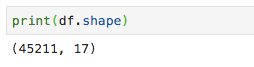The number of rows and columns would be printed in the output.

The next thing to do is to examine each column's suitability for the model that we are trying to build.

# Restructuring Data

Whenever an organization conducts a survey, they try to collect as much information as possible from the customer, with the idea that it would be useful to the organization one way or the other someday. To solve the problem at our hands, we have to pick up the information directly relevant to our problem.

## Displaying All Fields

Now, let us see how to select the data fields useful to us. Run the following statement in the code editor.

``````print(list(df.columns))
``````

You will see the following output: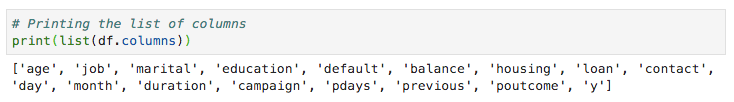The output shows the names of all the columns in the database. The last column “y” is a column indicating whether this customer has a TD. The values of this field are either “yes” or “no.” You can read each column’s description and purpose in the banks-name.txt file that was downloaded as part of the data.

## Eliminating Unwanted Fields

Examining the column names, you will know that some of the fields have no significance to the problem. For example, fields such as a month, day_of_week, campaign, etc. are of no use. We will eliminate these fields from our database. To drop a column, we use the drop command, as shown below:

``````#drop columns which are not needed.
df.drop(df.columns[[0, 3, 5, 8, 9, 10, 11, 12, 13, 14]], axis=1, inplace=True)
``````

The command says that drop column numbers 0, 3, 5, 8, and so on. To ensure that the index is properly selected, use the following statement: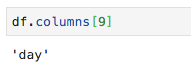This prints out the column name for the given index.

After dropping the columns which are not required, examine the data with the head statement. The screen output is shown here: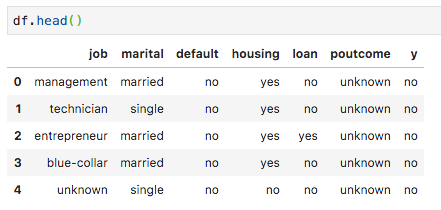Now, we have only the fields which we feel are essential for our data analysis and prediction. Here comes the importance of a Data Scientist. The data scientist has to select the appropriate columns for model building. For example, the type of job, though at first glance, may not convince everybody for inclusion in the database, it will be a very useful field. Not all types of customers will open the TD. The lower-income people may not open the TDs, while the higher-income people will usually park their excess money in TDs. So, the type of job becomes significantly relevant in this scenario. Likewise, carefully select the columns which you feel will be suitable for your analysis.

Next, we will prepare our data for building the model.

## Preparing Data

For creating the classifier, we must preprocess the data in a format that is asked by the classifier building module. We prepare the data by doing One Hot Encoding.

### Encoding Data

I will explain what we mean by encoding data shortly. First, let us run the code. Run the following command in the code window.

``````# creating one hot encoding of the categorical columns.
data = pd.get_dummies(df, columns =['job', 'marital', 'default', 'housing', 'loan', 'poutcome'])
``````

As the comment says, the above statement will create the one hot encoding of the data. Let us see what it has created. Examine the generated data called “data” by printing the head records in the database.

``````data.head()
``````

You will see the following output: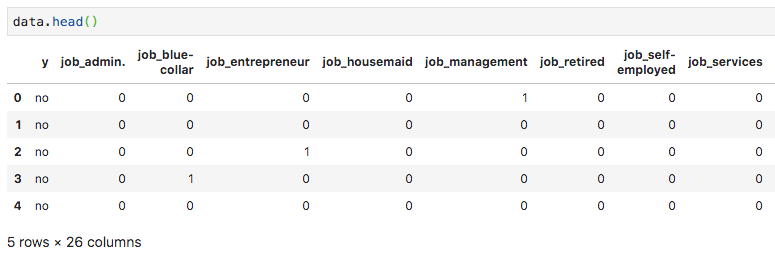To understand the above data, we will list out the column names by running the `data.columns` command, as shown below: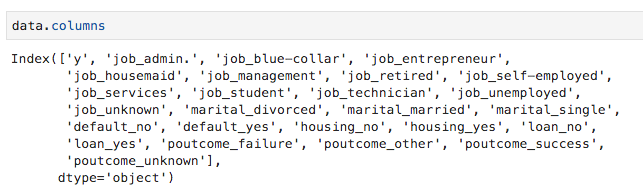Now, I will explain to you how the get_dummies command does the one-hot encoding. The first column in the newly generated database is the “y” field, which indicates whether this client has subscribed to a TD or not. Now, let us look at the columns which are encoded. The first encoded column is “job.” In the database, you will find that the “job” column has many possible values such as “admin,” “blue-collar,” “entrepreneur,” and so on. For each possible value, we have a new column created in the database, with the column name appended as a prefix. Thus, we have columns called “job_admin,” “job_blue-collar,” and so on. For each encoded field in our original database, you will find a list of columns added in the created database with all possible values that the column takes in the original database. Carefully examine the list of columns to understand how the data is mapped to a new database.

## Understanding Data Mapping

To understand the generated data, let us print out the entire data using the data command. The partial output after running the command is shown below.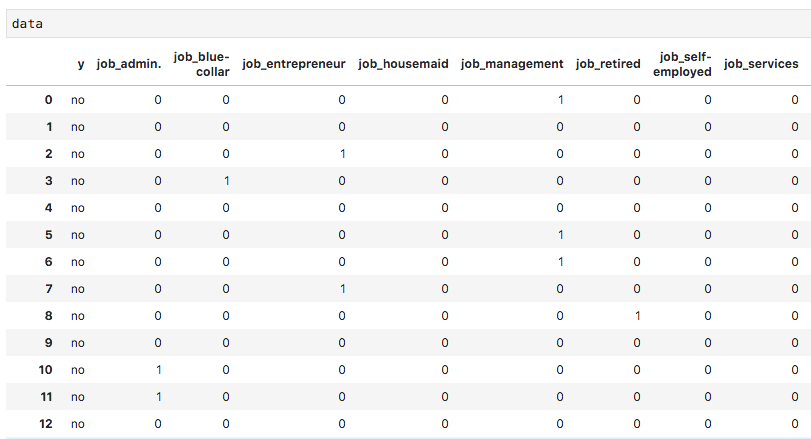The above screen shows the first twelve rows. If you scroll down a little bit, you see that the mapping is done for all the rows. A partial screen output further down the database is shown here for your quick perusal.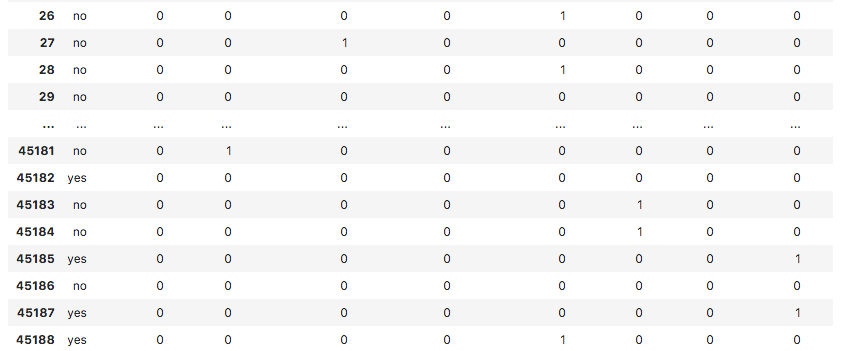To understand the mapped data, let us examine the first row.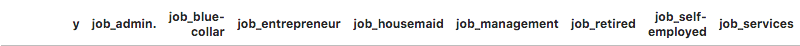It says that this customer has not subscribed to TD, as indicated by the value in the “y” field. It also shows that this customer is a “management” customer. Scrolling down horizontally, it will tell you that he has a “housing” and has taken no “loan.”

After this one-hot encoding, we need some more data processing before we can start building our model.

## Dropping the “unknown”

If we examine the columns in the mapped database, you will find few columns ending with “unknown.” For example, examine column at index 12 with the following command shown in the screenshot: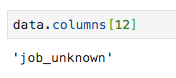This indicates the job for the specified customer is unknown. There is no point in including such columns in our analysis and model building. Thus, all columns with the “unknown” value should be dropped. This is done with the following command:

``````data.drop(data.columns[[12, 25]], axis=1, inplace=True)
``````

Ensure that you specify the correct column numbers. In case of a doubt, you can anytime examine the column name by specifying its index in the columns command as described earlier.

After dropping the undesired columns, you can examine the final list of columns as shown in the screenshot below: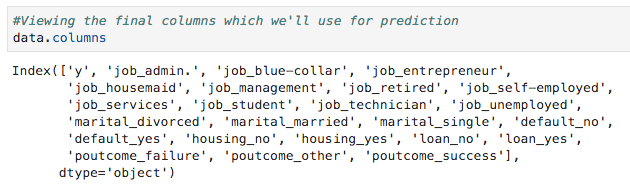At this point, our data is ready for model building.

## Splitting Data

We have about forty-one thousand and odd records. If we use the entire data for model building, we will not be left with any data for testing. So generally, we split the entire data set into two parts, say 70/30 percentage. We use 70% of the data for model building and the rest for testing the accuracy in the prediction of our created model. You may use a different splitting ratio as per your requirement.

## Creating Features Array

Before we split the data, we separate the data into two arrays X and Y. The X array contains all the features that we want to analyze, and Y array is a single-dimensional array of boolean values that is the output of the prediction. To understand this, let us run some code. Execute the following Python statement to create the X array:

``````X = data.iloc[:,1:]
``````

To examine the contents of X, use the head to print a few initial records. The following screen shows the contents of the X array.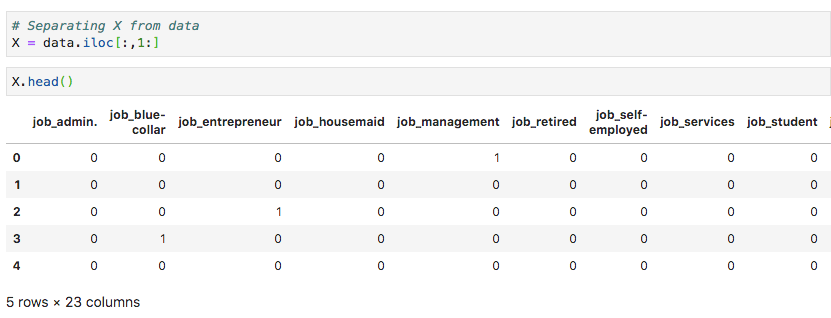The array has several rows and 23 columns.

Next, we will create an output array containing “y” values.

## Creating Output Array

To create an array for the predicted value column, use the following Python statement:

``````Y = data.iloc[:,0]
``````

Examine its contents by calling head. The screen output below shows the result: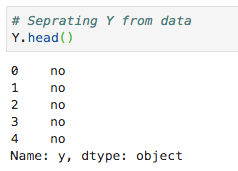Now, split the data using the following command:

X_train, X_test, Y_train, Y_test = train_test_split(X, Y, random_state=0)

This command will create the four arrays called X_train, Y_train, X_test, and Y_test. As before, you may examine the contents of these arrays by using the head command. We will use X_train and Y_train arrays for training our model and X_test and Y_test arrays for testing and validating.

Now, we are ready to build our classifier.

## Building Classifier

Fortunately, we do not have to build the classifier from scratch. Building the classifier from scratch is the subject of a student learning Computer Science. Building classifiers is complex and requires knowledge in several areas such as statistics, probability theories, optimization techniques, and so on. There are several pre-built libraries available in the market which have a fully-tested and very efficient implementation of these classifiers. We will use one such pre-built model from the sklearn.

### The sklearn Classifier

Creating the Logistic Regression classifier from sklearn toolkit is trivial and is done in a single program statement as shown here:

``````classifier = LogisticRegression(solver='lbfgs',random_state=0)
``````

The solver parameter determines the optimization algorithm that needs to be used. There are several algorithms available for optimization. The lbfgs is the default value for this parameter, so it need not be included in the call. I just included it to make it more explicit to you. Once the classifier is created, you will feed your training data into the classifier so that it can tune its internal parameters and be ready for the predictions on your future data. To tune the classifier, we run the following statement:

``````classifier.fit(X_train, Y_train)
``````

The classifier is now ready for testing. The following screen shows the output of the execution of the above two statements: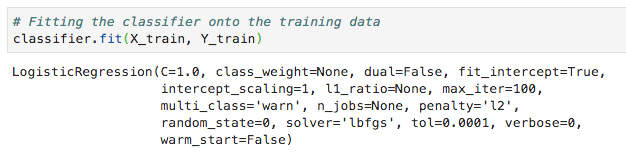Now, we are ready to test the created classifier.

## Testing

We need to test the above-created classifier before we put it into production use. If the testing reveals that the model does not meet the desired accuracy, we will have to go back in the above process, select another set of features (data fields), build it again, and test it. This will be an iterative step until the classifier meets your requirement of the desired accuracy. So, let us test our classifier.

### Predicting Test Data

To test the classifier, we use the test data generated in the earlier stage. We call the predict method on the created object and pass the X array of the test data, as shown in the following command:

``````predicted_y = classifier.predict(X_test)
``````

This command generates a single dimensional array for the entire training data set, giving each row's prediction in the X array. Examine this array by using the following command:

``````predicted_y
``````

The following screen shows the output of executing the above two commands: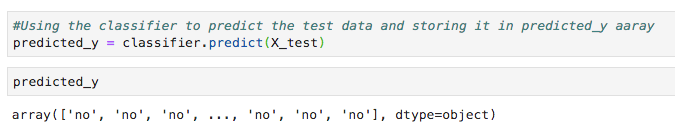The output indicates that the first and last three customers are not the potential candidates for the Term Deposit. You can examine the entire array to sort out potential customers. To do so, use the following Python code snippet:

``````for x in range(len(predicted_y)):
if (predicted_y[x] == “yes”):
print(x, end="\t")
``````

The output of running the above code is shown below: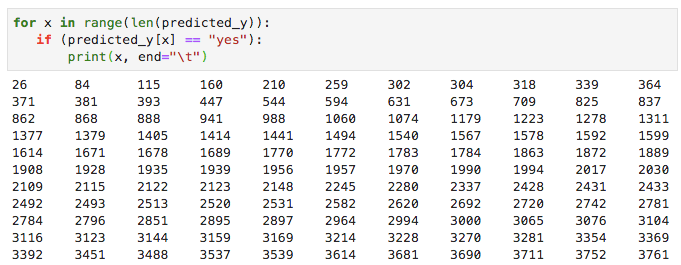The output shows the indexes of all rows, which are probable candidates for subscribing to TD. You can now give this output to the bank’s marketing team, who would pick up the contact details for each customer in the selected row and proceed with their job.

Before we put this model into production, we need to verify the accuracy of the prediction.

## Verifying Accuracy

To test the accuracy of the model, use the score method on the classifier, as shown below:

``````print('Accuracy: {:.2f}'.format(classifier.score(X_test, Y_test)))
``````

The screen output of running this command is shown below: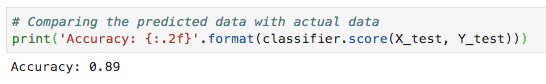It shows that the accuracy of our model is 90%, which is considered very good in most of the applications. Thus, no further tuning is required. Now, our customer is ready to run the next campaign, get the list of potential customers, and chase them for opening the TD with a probable high rate of success.

## Limitations

As you have seen from the above example, applying logistic regression for machine learning is not difficult. However, it comes with its limitations. The logistic regression will not be able to handle a large number of categorical features. In our example, we reduced the number of features to a considerable extent. However, if these features were important in our prediction, we would have been forced to include them, but then, the logistic regression would fail to give us a good accuracy. Logistic regression is also vulnerable to overfitting. It cannot be applied to a non-linear problem. It will perform poorly with independent variables that are not correlated to the target and are correlated to each other. Thus, you will have to carefully evaluate the suitability of logistic regression to the problem that you are trying to solve.

There are many areas of machine learning, where other techniques are specially devised. To name a few, we have algorithms such as k-nearest neighbors (kNN), Linear Regression, Support Vector Machines (SVM), Decision Trees, Naive Bayes, and so on. Before freezing on a particular model, you will have to evaluate the applicability of these various techniques to the problem that you are trying to solve.

# Summary

Logistic Regression is a statistical technique of binary classification. In this tutorial, you learned how to train the machine to use Logistic Regression. While creating machine learning models, the most crucial requirement is the availability of the data. Without adequate and relevant data, you cannot simply make the machine to learn. Once you have data, your next major task is cleansing the data, eliminating the unwanted rows, fields, and selecting the appropriate fields for your model development. After this is done, you need to map the data into a format that your classifier demands training. So data preparation is a significant task in any machine learning application. Once you are ready with the data, you can select a particular type of classifier. In this tutorial, you learned how to use a logistic regression classifier provided in the sklearn library. To train the classifier, we use about 70% of the data for training the model. We use the rest of the data for testing. We test the accuracy of the model. If this is not within acceptable limits, we go back to selecting the new set of features. Once again, follow the entire process of preparing data, train the model, and test it until you are satisfied. Before taking up any machine learning project, you must learn and have exposure to a wide variety of techniques that have been developed so far and have been applied successfully in the industry.